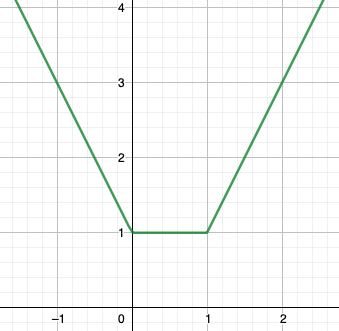# Does a convex function only have one minimum?

## Question:

Does a convex function only have one minimum?

## Convex and Strictly Convex Functions:

Suppose that {eq}f(x) {/eq} is a function defined on an interval {eq}I {/eq}. Then we say that:

• {eq}f(x) {/eq} is a convex function if, for any {eq}a {/eq} and {eq}b {/eq} in {eq}I {/eq} and any {eq}t {/eq} in {eq}(0,1) {/eq}, {eq}f(x) {/eq} satisfies the inequality {eq}f\big((1-t)a+tb\big) \le (1-t)f(a)+tf(b) {/eq}.
• {eq}f(x) {/eq} is a strictly convex function if, for any {eq}a {/eq} and {eq}b {/eq} in {eq}I {/eq} with {eq}a \ne b {/eq} and any {eq}t {/eq} in {eq}(0,1) {/eq}, {eq}f(x) {/eq} satisfies the inequality {eq}f\big((1-t)a+tb\big) < (1-t)f(a)+tf(b) {/eq}.

Informally, {eq}f {/eq} is convex if the line segment joining {eq}(a,f(a)) {/eq} and {eq}(b,f(b)) {/eq} never drops below the graph of {eq}y=f(x) {/eq}, and strictly convex if additionally that line segment only intersects the graph of {eq}y=f(x) {/eq} at its endpoints.

Let {eq}f(x)=|x|+|x-1| {/eq}. Then {eq}f(x) {/eq} is the sum of the convex functions {eq}|x| {/eq} and {eq}|x-1| {/eq}, so {eq}f(x) {/eq} is convex. The graph of {eq}f(x) {/eq} is shown below:As you can see, {eq}f(x)=1 {/eq} whenever {eq}0 \le x \le 1 {/eq}. We can see that this is the minimum value of {eq}f(x) {/eq} as follows:

{eq}\begin{align*} f(x)&=|x|+|x-1|\\ &=|x|+|1-x|&&\text{(since the absolute value function is even)}\\ &\ge |x+1-x|&&\text{(by the triangle inequality)}\\ &=1 \, . \end{align*} {/eq}

So {eq}f(x) \ge 1 {/eq} for all {eq}x {/eq}, which means that any point {eq}x {/eq} in {eq}[0,1] {/eq} is a minimum for {eq}f(x) {/eq}. That is, a convex function need not have only one minimum.

On the other hand, a strictly convex function will always have at most one minimum. To see this, suppose that {eq}g(x) {/eq} is strictly convex, and {eq}g(a)=g(b)=m {/eq}. Then for any {eq}x {/eq} in {eq}(a,b) {/eq}, there is some {eq}t {/eq} in {eq}(0,1) {/eq} such that {eq}x=(1-t)a+tb {/eq}. We therefore have

{eq}\begin{align*} g(x)&=g\big((1-t)a+tb\big)\\ &<(1-t)g(a)+tg(b)&&\text{(because }g\text{ is strictly convex)}\\ &=(1-t)m+tm&&\text{(since }g(a)=g(b)=m\text{ as defined above)}\\ &=m-tm+tm&&\text{(expanding)}\\ &=m&&\text{(canceling opposite terms).} \end{align*} {/eq}

That is, {eq}g(x)<m {/eq} for any {eq}x {/eq} in {eq}(a,b) {/eq}, and so {eq}m=g(a)=g(b) {/eq} cannot be the minimum value of {eq}g {/eq}.

In summary, we've shown that if a strictly convex function {eq}g {/eq} takes on the same value at any two distinct points, then that value is not the minimum value of {eq}g {/eq}. This means that {eq}g {/eq} can have at most one minimum.